#Quality Digest

Featured Product
This Week in Quality Digest Live
Statistics Features
William A. Levinson
People can draw the wrong conclusions due to survivor, survey, and bad news bias.
Donald J. Wheeler
Does your approach do what you need?
Paul Laughlin
Correlation vs. causality
Donald J. Wheeler
In spite of what everyone says to the contrary
Donald J. Wheeler
What can you say about the sampled lot?
Statistics News
Elsmar Cove is a leading forum for quality and standards compliance
InfinityQS’ quality solutions have helped cold food and beverage manufacturers around the world optimize quality and safety
User friendly graphical user interface makes the R-based statistical engine easily accessible to anyone
Collect measurements, visual defect information, simple Go/No-Go situations from any online device
Good quality is adding an average of 11 percent to organizations’ revenue growth
Ability to subscribe with single-user minimum, floating license, and no long-term commitment
A guide for practitioners and managers
Gain visibility into real-time quality data to improve manufacturing process efficiency, quality, and profits
Tool for nonstatisticians automatically generates models that glean insights from complex data setsBio

Statistics

## So What Is the Fraction Nonconforming?

### How to know what the data are really telling you

Published: Monday, November 8, 2021 - 13:03

One of the most common questions about any production process is, “What is the fraction nonconforming?” Many different approaches have been used to answer this question. This article will compare the two most widely used approaches and define the essential uncertainty inherent for all of these approaches.

In order to make the following discussion concrete we will need an example. Here we shall use a collection of 100 observations obtained from a predictable process. These values are the lengths of pieces of wire with a spade connector on each end. These wires were used to connect the horn button on a steering wheel assembly. For purposes of our discussion let us assume the upper specification for this length is 113 mm.Figure 1: Histogram of 100 wire lengths

### The empirical approach

The oldest and simplest approach to estimating the fraction nonconforming is arguably the best approach. We simply divide the number of items that are outside the specifications, Y, by the total number of items inspected, n. The name commonly used for this ratio is the binomial point estimate: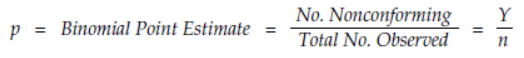This ratio provides an unbiased estimate of the process fraction nonconforming. For the data of figure 1, six of the observed values exceed 113, so Y = 6 while n = 100, and our binomial point estimate for the fraction nonconforming is p = 0.06 or 6 percent.

(Note that calling the ratio above the binomial point estimate is simply a label. It identifies the formula. If the two counts above satisfy certain conditions then this ratio would provide a point estimate of a binomial parameter. Calling the ratio the binomial point estimate does not imply any assumption about a probability model for either the counts or the measurements upon which they are based.)

If the data come from 100-percent inspection, then there is no uncertainty in the descriptive ratio above. The 6 percent is the fraction rejected at inspection, and the only uncertainty is the uncertainty of an item being misclassified.

However, if we are using the data of figure 1 to represent product not measured, or to predict what might be made in the future, then we will need to be concerned with the uncertainty involved in the extrapolation from the product measured to the product not measured. Here, because the production process was being operated predictably, this extrapolation makes sense.

When data are used for representation or prediction we will need to use an interval estimate in addition to the point estimate for the fraction nonconforming. The interval estimate will define the range of values for the process fraction nonconforming that are consistent with the observed point estimate.

Most textbooks will give a formula for an approximate 95-percent interval estimate that is centered on the binomial point estimate p.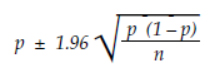This formula is commonly referred to as the Wald interval estimate (even though it was first published by Pierre Simon Laplace in 1812). While this simple approximation is satisfactory when the proportions are in the middle of the range between 0.00 and 1.00, it does not work well for proportions that are close to either 0.00 or 1.00. Since the fraction nonconforming will hopefully be near 0.00, we will need to use the more robust Agresti-Coull interval estimate.

The 95-percent Agresti-Coull interval estimate uses a formula similar to the Wald formula above, but it uses the Wilson point estimate in that formula. For a 95-percent interval estimate the Wilson point estimate is approximated by adding two successes and two failures: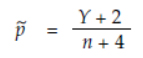With this adjustment we obtain a 95-percent interval estimate that works all the way down to Y = 0. In our example, using Y = 6 and n = 100, the Wilson point estimate is 0.0769, and the 95-percent Agresti-Coull interval estimate for the process fraction nonconforming is: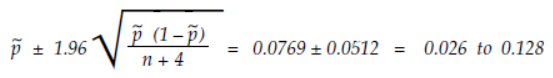So, the data in figure 1 give us a binomial point estimate of 6 percent, and a 95-percent Agresti-Coull interval estimate for the process fraction nonconforming of 2.6 percent to 12.8 percent. While 6 percent nonconforming is our best point estimate, the uncertainty of the extrapolation from our observed values to the underlying process means that our observed value of 6 percent nonconforming is consistent with a process that is producing anywhere from 2.6 percent to 12.8 percent nonconforming.

If we changed the upper specification for our example to be 114 mm, then Y would be 3, the binomial point estimate would be 3 percent, and the 95-percent Agresti-Coull interval estimate would be 0.0481 ± 0.0411. Thus, an observed value of 3 percent nonconforming would be consistent with a process fraction nonconforming between 0.7 percent and 8.9 percent.

Now consider what would happen if the upper specification was 116 mm. Here Y would be 0, the binomial point estimate would be 0 percent, and yet the 95-percent Agresti-Coull interval estimate would be 0.0192 ± 0.0264. Thus, our observed value of 0.0 percent nonconforming would be consistent with a process fraction nonconforming between 0.0 percent and 4.6 percent.

Since Y cannot get any smaller than zero, this last interval estimate reflects the limitations of the inferences that can be drawn from 100 observed values. Processes producing less than 4.6 percent nonconforming can, and will, produce some 100 piece samples that have zero nonconforming items!

Thus, the Agresti-Coull interval estimate provides us with a way to characterize the process fraction nonconforming based on the observed data. It defines the uncertainty that is inherent in any use of the data to estimate the process fraction nonconforming.

### The probability model approach

Sometimes, rather than using the data to directly estimate the fraction nonconforming, a probability model is fitted to the histogram and used to compute the tail areas beyond the specification limits. While the data are used in fitting the probability model to the histogram, the estimate of the fraction nonconforming will be obtained from the fitted model rather than directly from the data. As an example of this approach we will fit a normal distribution to the wire length data.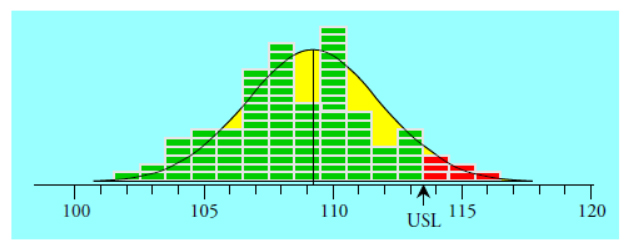Figure 2: A normal distribution fitted to the wire length data

The wire length data have an average of 109.19 mm, a standard deviation statistic of 2.82 mm, and the process behavior chart shows no evidence of unpredictable operation while these values were obtained. A normal probability model having a mean of 109.19 and a standard deviation parameter of 2.82 is shown superimposed on the wire length data in figure 2.

As before, we assume that the upper specification limit is 113 mm. Since the measurements were made to the nearest whole millimeter, this upper spec becomes 113.5 mm in the continuum used by the model. When we standardize 113.5 mm we obtain a z-score of 1.53, and from our standard normal table we find that this corresponds to an upper tail area of 0.0630. Thus, using a normal probability model we obtain a point estimate of the process fraction nonconforming of 6.3 percent, which is essentially the same as the binomial point estimate found earlier.

So, just how much uncertainty is attached to this estimate? Judging from the few cases where the interval estimate formulas are known for model-based point estimates, we can say that if the probability model is appropriate, then this estimate is likely to have a slightly smaller interval estimate than the empirical approach. However, if the probability model is not appropriate, then this estimate can have substantially more uncertainty than the empirical estimate. Which brings us to the first problem with using a probability model: Any choice of a probability model will, in the end, turn out to be an unverifiable assumption. It amounts to nothing more than an assertion made by the investigator.

While lack-of-fit tests may sometimes allow us to rule out a probability model, no test will ever allow us to validate a particular probability model. Moreover, given a sufficient amount of data, you will always detect a lack of fit between your data and any probability model you may choose. This inability to validate a model is the reason that it is traditional to use the normal distribution when converting capabilities into fractions nonconforming. Since the normal distribution is a maximum entropy distribution, its use amounts to performing a generic, worst-case analysis. (It is important to note that this use of a normal distribution is a matter of convenience, arising out of a lack of information, and is not the same as an a priori requirement that the data “be normally distributed.”)

To illustrate the generic nature of estimates based on the normal distribution we will use the ball-joint socket thickness data shown in figure 3. There we have 96 values collected over the course of one week while the process was operated predictably. The average is 4.656 and the standard deviation statistic is 1.868.

The first probability model fitted to these data is a normal distribution having a mean of 4.656 and a standard deviation parameter of 1.868. There is a detectable lack of fit between the histogram and this normal distribution.

The second probability model fitted to these data is a gamma distribution with alpha = 6.213 and beta = 0.749. This model has a mean if 4.654 and a standard deviation of 1.867. There is no detectable lack of fit between this gamma distribution and the histogram.

The third probability model fitted to these data is a Burr distribution with c = 1.55 and k = 58.55 that has been shifted to have a mean of 4.656 and stretched to have a standard deviation parameter of 1.868. There is no detectable lack of fit between this Burr distribution and the histogram.

So we have two models that “fit” these data and one model that “does not fit” these data.Figure 3: Three probability models fitted to the socket thickness data

Skewed histograms usually occur when the data pile up against a barrier or boundary condition. As a result we are commonly concerned with the areas in the elongated tail. So here we will consider the upper tail areas defined by the cutoff values of 5.5, 6.5, 7.5, etc. Figure 4 shows these upper tail areas computed four ways: (1) using the normal distribution, (2) using the fitted gamma distribution, (3) using the fitted Burr distribution, and (4) using the empirical binomial point estimate. In addition, we find that the 95-percent Agresti-Coull intervals bracket all four estimates for each cutoff value.Figure 4: Four different point estimates of upper tail areas for the socket thickness data

In spite of the differences between the four estimates in each row, all four estimates fall within the 95-percent Agresti-Coull interval. This illustrates what will generally be the case: The uncertainty inherent in the data will usually be greater than the differences between the various model-based estimates.

This makes any discussion about which model-based estimate is best into an argument about noise. When we are estimating the fraction nonconforming, the uncertainty in our estimates will generally overwhelm the differences due to our choice of probability model. This uncertainty even covered the estimates when there was a detectable lack of fit for the normal distribution.

The numbers you obtain from a probability model are never really as precise as they look.

This is why the generic ballpark values obtained by using a normal distribution are generally sufficient. The ballpark is so large that the normal distribution will get you in the right neighborhood even when there is a detectable lack of fit.

“But using a fitted probability model will let us compute tail areas for capability indexes greater than 1.00.”

Yes, it will, and that is the second problem with the probability model approach. No matter how many data you have, there will always be a discrepancy between the extreme tails of your probability model and the tails of your histogram. This happens simply because histograms always have finite tails.

### How histograms grow with n

Figure 5 shows the average number of standard deviations between the average value for a histogram and the most extreme value of that histogram. As a histogram grows to include more data the maximum and minimum values move away from the average value. Figure 5 shows the average size of the finite tails of histograms involving different amounts of data.Figure 5: Average number of standard deviations between the average and the most extreme value for histograms of n data

Once you get beyond 200 data, the tails of the histogram grow ever more slowly with the increasing number of data. While most of the values in figure 5 have been known since 1925, this aspect of data analysis has seldom been taught to our students. Histograms with less than 1,000 data will rarely have points more than 3.3 standard deviations away from the average. This means that the major discrepancies between a probability model and a histogram are going to occur in the region out beyond three standard deviations on either side of the mean.

These discrepancies undermine all attempts to use probability models to compute meaningful fractions nonconforming when the capability indexes get larger than 1.10. The values in figure 5 show that when the capability indexes get larger than 1.10 you will commonly have no data points outside the specifications. As a result your count Y will generally be zero, the point binomial estimate will be zero, and the Agresti-Coull interval estimate will depend solely upon the number of data in the histogram.

However, when we use a probability model to estimate the fraction nonconforming for capability indexes larger than 1.00 we will have to compute infinitesimal areas under the extreme tails of the assumed probability model. Here the result will depend upon our assumption rather than depending upon the data.

To illustrate this dependence figure 6 will extend figure 4 to cutoff values beyond three sigma. Here the upper tail areas are given in parts per million.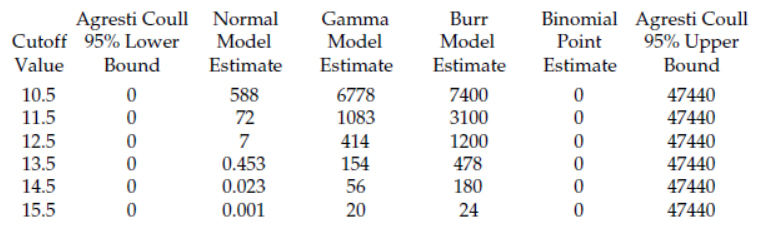Figure 6: Additional upper tail areas for the socket thickness data in parts per million

Both the gamma model and the Burr model showed no detectable lack of fit with these data. Yet the upper tail areas from these two “fitted” models differ by as much as a factor of three. So, which model is right?

Say the upper specification limit for the socket thickness data is 13.5. Which estimate from figure 6 should you tell your boss?
1. One-half part per million nonconforming?
2. One hundred fifty-four parts per million nonconforming?
3. Four hundred seventy-eight parts per million nonconforming?
4. Or something less than 4.7 percent nonconforming?

Only the fourth answer is based on the data. The first three values are imaginary answers based on the infinitesimal areas in the extreme tails of assumed probability models.

When the uncertainty interval is zero to 47,000 parts per million, any model you pick, regardless of whether or not it “fits the data,” will manage to deliver an estimate that falls within this interval.

So while our assumed probability models allow us to compute numbers out to parts per million and even parts per billion, these data will not support an estimate that is more precise than something less than five parts per hundred. Think about this very carefully.

When you compute tail areas out beyond three sigma you are computing values that are entirely dependent upon the assumed probability model. These tail areas will have virtually no connection to the original data. Because of the inherent discrepancy between the tails of the histogram and the tails of the probability model, the conversion of capability indexes that are larger than 1.00 into fractions nonconforming will tell you more about the assumed model than it will tell you about either the data or the underlying process. This is why such conversions are complete nonsense.

### Summary for model-based estimates

Thus, there are two problems with using a probability model to estimate the process fraction nonconforming. The first is that any choice of a probability model is essentially arbitrary, and the second is that the use of a probability model encourages you to extrapolate beyond the tails of the histogram to compute imaginary quantities.

Given the uncertainty attached to any estimate of the process fraction nonconforming, the choice of a probability model will usually make no real difference as long as the capability indexes are less than 1.00. Here the use of a generic normal distribution will provide reasonable ballpark values, and there is little reason to use any other probability model.

However, when the specifications fall beyond the tails of the histogram, and especially when the capability indexes exceed 1.10, no probability model will provide credible estimates of the process fraction nonconforming. Computing an infinitesimal area under the extreme tails of an assumed probability model is an exercise that simply has no contact with reality.

### So what do we really know?

What we know depends upon how many data we have and whether or not those values were collected while the process was operated predictably. Moreover, the only way to determine if the data were obtained while the process was operated predictably is by using a process behavior chart with rational sampling and rational subgrouping.

If the data show evidence that the process was changing while the data were collected, then the process fraction nonconforming may well have also changed, making any attempt at estimation moot. (In the absence of a reasonable degree of predictability, all estimation is futile.)

If the data show no evidence of unpredictable operation, then we may use the binomial point estimate to characterize the process fraction nonconforming. In addition we may also use the 95-percent Agresti-Coull interval estimate to characterize the uncertainty in our point estimate. This approach is quick, easy, robust, and assumption free.

When no observed values fall beyond a specification limit our count Y becomes zero and the binomial point estimate goes to zero. However, the 95-percent Agresti-Coull interval estimate will still provide an upper bound on the process fraction nonconforming. These upper bounds will depend solely upon the number of observations in the histogram, n. Selected values are shown in figure 7.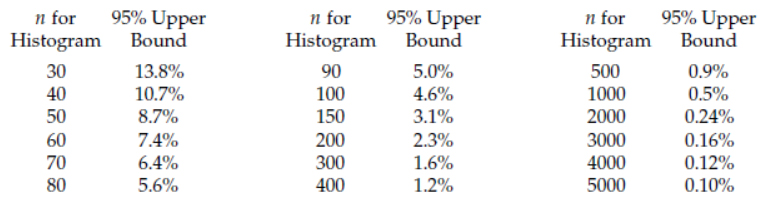Figure 7: The uncertainty in estimates of process fraction nonconforming when Y = 0

The upper bounds listed in figure 7 define the essential uncertainty in ALL estimates of the fraction nonconforming that correspond to a count of Y = 0 nonconforming. This means that when you use a probability model to compute a tail area that is beyond the maximum or the minimum of your histogram, then regardless of the size of your computed tail area, the process fraction nonconforming can be anything up to the upper bound listed in figure 7. There we see that it takes more than 1,000 data to get beyond the parts per hundred level of uncertainty.

Say, for example, that you have a histogram of 70 data collected while the process was operated predictably and on target with an estimated capability ratio of 1.33. Say that these data are suitably bell-shaped and that you use a normal distribution to estimate the process fraction nonconforming to be 64 parts per million. Figure 7 tells us that with only 70 data all you really know about the process fraction nonconforming is that it is probably less than 6.4 percent nonconforming. This is 1,000 times greater than the computed value of 64 ppm! With this amount of uncertainty how dogmatic should you be in asserting that the process fraction nonconforming is 64 parts per million?

Until you have hundreds of thousands of data collected while the process is operated predictably you simply do not have any basis for claiming that you can estimate the fraction nonconforming to the parts-per-million level.

One day a client from a telephone company observed that they computed the number of dropped calls each day in parts per million. However, in this case they were using the empirical approach and the denominator was in the tens of millions. With this amount of data the uncertainty in the computed ratio was less than 0.5 ppm, and reporting this number to the parts-per-million level was appropriate.

But for the rest of you, those who have been using a few dozen data, or even a few hundred data, as the basis for computing parts-per-million nonconforming levels, I have to tell you that the numbers you have been using are no more substantial than a mirage. The uncertainties in such numbers are hundreds or thousands of times larger than the numbers themselves.

The first lesson in statistics is that all statistics vary. Until you understand this variation you will not know the limitations of your computed values. Using parts per million numbers based on probability models fitted to histograms of a few hundred data without regard to their uncertainties is a violation of this first lesson of statistics. It is a sign of a lack of understanding regarding statistical computations. Hopefully, now you know how to estimate the fraction nonconforming and how to avoid some of the snake oil that is out there.

### Discuss### Donald J. Wheeler

Find out about Dr. Wheeler’s virtual seminars for 2022 at www.spcpress.com. Dr. Wheeler is a fellow of both the American Statistical Association and the American Society for Quality who has taught more than 1,000 seminars in 17 countries on six continents. He welcomes your questions; you can contact him at djwheeler@spcpress.com.

### So What is the fraction Nonconforming

Is there a citation for the Agresti-Coull  equation?  Curious about where they get the 2 and the 4.

### Citation

Here's a citation for you, anonymous: Agresti, A., & Coull, B. A. (1998). Approximate is better than “exact” for interval estimation of binomial proportions. The American Statistician, 52(2), 119-126.

Don, I have to ask, though...why n+4 if we're adding 2 to the failures and 2 to the successes? I understand Y+2, but why n+4? To adjust when you take the square root?

The Agresti-Coull confidence interval uses the Wilson point estimate. The Wilson point estimate adds the z-score for the alpha level to both the number of successes and the number of failures.  So, for a 95% interval we add 1.96 successes and 1.96 failures to the counts.  Since we are dealing with counts we typiclaly round these off to 2 successes and 2 failures.  For a 90% Agresti Coull interval the Wilson point estimate would add 1.65 failures and 1.65 successes, etc.  The Wilson point estimtes were used in yet another formula for a confidence interval, done in the first half of the 20th Century.  In 2003 Agresti and Coull did an extensive survey of all the ways of obtaining a confidence interval for a proportion, analyzed how they worked in practice, and came up with their synthesis that is now the preferred method for such a confidence interval.

### Another nail

When stepping back and forgetting everything about statistics for a moment, it is possible to see that the usual talk about parts per million becomes meaningless. Simply by looking at a histogram, you can tell that there is just no way that enough information is present in the samples to extrapolate to anything about parts per million.

Six-Sigma is sloppy with its parts per millions (and billions!) and has not bothered to carry the burden of evidence. Instead, the burden has been reversed and left to the critics. But disproving something is much harder than making claims and less glamorous, so I think this is important work from the author.

### Estimates

My critique of many Six Sigma authors is that they do not understand that any sample can only give you an estimate, and that estimates carry uncertainty. I have read (and don't ask for a citation...I read this years ago in an article) that if your process produces 6 parts per million defective, you have not reached "six sigma" quality!

In my view, a lot of this comes from rule 4 of the funnel. Jack Welch didn't know anything about statistics, but he knew how to sell Wall Street on what he thought was a great cost-cutting scheme. This did a number of things: Popularized Six Sigma in the CEO set, which created demand for Six Sigma consultants and trainers, which led many consulting firms (largely populated by accountants) to get on the bandwagon and develop programs that demanded projects that would yield \$250K or more (and rejected any that did not). At GE, engineers who felt that "It's better to have a sister in a cathouse than a brother in quality control" and "a camel is a horse designed by a team" and "the quickest way to ruin something is to improve it" were suddenly forced to become Black Belts. (The quotes, by the way, are from my father, a life-long GE engineer...when I told him what my new career aspirations were after going to a Deming seminar). So now you had a lot of Black Belts who were forced to go to training that did not interest them...some of them probably had projects that didn't make the 250K cut, and so essentially pulled 16 red beads, made the bottom 10% and ended up on the street. At that time, guess which bullet in their resume made them valuable? GE Black Belt. So now you have novices being trained by neophytes. Many of them ended up as consultants or trainers...

Add to this that statistical thinking is counterintuitive and not something that humans inherently do; they have to be taught, they have to practice, they have to want to learn and to care. Most people don't, and most Americans, taught statistics for enumerative studies, couldn't begin to understand Deming or Wheeler or Nelson. As engineers and accountants, they believed in the precision of numbers and the reliability of prediction.

So, it's OK to estimate a fraction non-conforming, but you have to remember it's an estimate. I have tried very hard in recent years to never, ever give a point estimate. I have adopted an approach I learned from Heero Haqueboord: when someone asks me for "the number," I tell them, "I'm pretty sure it's going to be somewhere between x and y." When they press me for a single number they can use for planning, I repeat, "I'm pretty sure it's..." They might get angry about it, but I usually end up telling them, "Look, I could give you one number, but it will be wrong. If anyone tells you that they are certain it is THIS number, run away from them and never listen to anything they say again."

### In favor of model-based estimate for a pharmaceutical process?

Operating in the pharmaceutical industry, is it not more realistic to report a model-based estimate of the process  nonconforming fraction? Assume a company makes 30 or 100 batches of a pharmaceutical  over a span of 5  or 10 months or years, all within-specifications (Y = 0).

Imagine what would be the reaction of a boss when informed that the nonconforming fraction of batches generated by a well-behaved predictable GMP-compliant manufacturing process could reach  13.8% and 4.6%, respectively? The Wald interval estimate and the Agresti-Coull limits are essentially based on n Bernoulli trials, in fact on a sampling experiment whereby n = 30 or n=100 batches are randomly selected from an infinite population of batches.

As we all know, data should be evaluated in their context. In reality, each batch is produced separately. Is it not preferable to adopt a simple practical approach: to collect the data, to characterize their distribution by fitting a reasonable model and then derive the nonconforming fraction?    Yes! The two disadvantages of the probability model mentioned by Dr. Wheeler (arbitrary probability model and data extrapolation) still hold. But, the outcome (a smaller nonconforming fraction) will be more consistent with the reality of zero nonconforming throughout repetitive manufacturing across a large span of time.

Yes! Statistics change. Given the information one has about his process at this point, one estimates the nonconforming fraction. As of today, the process generates zero and the expected nonconforming fraction is consequently small or very small. As more data are collected,  an updated nonconforming fraction wll be calculated and reported.  Isn't this practical approach preferable rather than reporting a worst-case value that appears non-consistent with the present behavior of the process?

To the best of my knowledge, the probability model is commonly applied in the pharmaceutical industry.

### Commonly applied =/= right

Being commonly applied is not the same thing as being right. It is certainly commonly applied in the medical device industry as well, so I'd venture that the same holds in pharma. But the whole point of this article is that this approach does not have enough data to produce an adequate level of certainty relative to the estimates. After getting 30 samples with zero failures, no one is claiming that the true failure rate is 4.6%, they are merely stating that we can't reduce the upper limit of certainty to anything below 4.6%. The true value might be 3.4 ppm, or 3.4 parts per thousand. No one can possibly know with such a limited amount of actual data. The probability model approach is just a way of lying to yourself about how precise the esitmate is. As Wheeler pointed out, there are many different probability models that you could fit and they will produce wildly different results. So why should you trust any specific one vs calling your upper limit essentially the worst case of any of the models you could possibly fit?

### Great point!

"The probability model approach is just a way of lying to yourself about how precise the estimate is."

That's an excellent way to sum it up. As to using the worst case out of all the models you could fit, Wheeler wrote some articles a few years ago arguing that the normal distribution is the distribution of maximum entropy.

I'm not always successful in this, but I always try to keep in mind a paragraph from Shewhart's Statistical Method from the Viewpoint of Quality Control:

"It must, however, be kept in mind that logically there is no necessary connection between such a physical statistical state and the infinitely expansible concept of a statistical state in terms of mathematical distribution theory. There is, of course, abundant evidence of close similarity if we do not question too critically what we mean by close. What is still more important in our present discussion is that if this similarity did not exist in general, and if we were forced to choose between the formal mathematical description and the physical description, I think we should need to look for a new mathematical description instead of a new physical description because the latter is apparently what we have to work with."